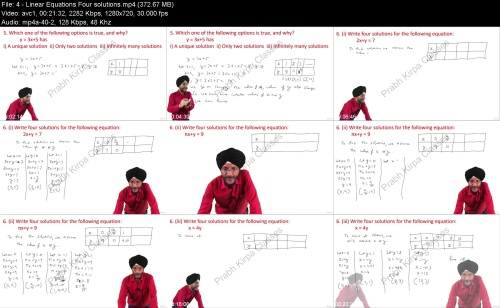# Linear Equation Part 2 Linear Equations in twoVariables

Posted in: Tutorials | By: AD-TEAM | 7-09-2023, 21:53 | 0 Comments
07
September
2023Linear Equation Part 2 Linear Equations in twoVariables
Language: English | Size:1.26 GB
Genre:eLearning

Files Included :

1 - Introduction.mp4 (199.19 MB)
MP4
2 - Expressing an equation as a Linear Equation.mp4 (329.69 MB)
MP4
3 - Linear Equations finding values of a b and c.mp4 (195.08 MB)
MP4
4 - Linear Equations Four solutions.mp4 (372.67 MB)
MP4
5 - Linear Equation Checking the given solutions.mp4 (193.3 MB)
MP4Note: